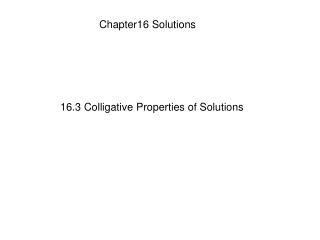Download PresentationChapter16 Solutions

# Chapter16 Solutions - PowerPoint PPT PresentationDownload Presentation## Chapter16 Solutions

- - - - - - - - - - - - - - - - - - - - - - - - - - - E N D - - - - - - - - - - - - - - - - - - - - - - - - - - -
##### Presentation Transcript

1. Chapter16 Solutions 16.3 Colligative Properties of Solutions

2. Chemistry Today we are learning to:-1. Identify threecolligative properties of a solution 2. Explain why vapor pressure, freezing point and boiling points of solutions differ from those of the pure solvent

3. Colligative Properties • Acolligative property a solution is just a property that depends on the number of particles in it. • Three important colligative properties of solutions are • vapor-pressure lowering • boiling-point elevation • freezing-point depression

4. Colligative Properties • vapor-pressure lowering • This is proportional to the number of solute particles dissolved in the solution. (more particles lower v.p.) • boiling-point elevation • This is proportional to the number of solute particles dissolved in the solution. (more particles higher b.p.) • freezing-point depression • This is proportional to the number of solute particles dissolved in the solvent. (more particles lower m.p.)

5. Colligative Properties • Why do solute particles have this effect? Intermolecular forces and ionic charges attract water molecules to the solute particles. This reduces the number of particles that have enough energy to vaporize.

6. Colligative Properties • Remember a colligative property a solution is just a property that depends on the number of particles in it. • So which of these will lower the freezing point of water the most: • 1 mole of NaCl (sodium chloride) • 0.5 mole of NaCl (sodium chloride) • They both lower it by the same amount • Which of these will lower the freezing point of water the most: • 1 mole of NaCl (sodium chloride) • 1mole of CaCl2 (calcium chloride) • They both lower it by the same amount Forms Na+ and Cl- = 2 ions Forms Na+ and 2Cl- = 3 ions

7. Colligative Properties

8. Colligative Properties • 1 mole of dissolved particles lowers the freezing point of 1000g of water by 1.86°C • 1 mole of dissolved particles elevates the boiling point of 1000g of water by 0.56°C

9. 16.3 Section Quiz. • Which of the following is NOT a colligative property • vapor-pressure lowering • freezing-point depression • boiling-point elevation • solubility elevation

10. 16.3 Section Quiz. 2. Choose the correct word for the space: The magnitude of each colligative property of solutions is proportional to the __________ solute dissolved in the solution. • type of • number of particles of • molar volume of • particle size of the

11. 16.3 Section Quiz. 3. The decrease in vapor pressure when a solute is added to a liquid is due to • attractive forces between solvent particles. • repulsion of the solute particles by the solvent particles. • dissociation of the solvent particles. • attractive forces between solvent and solute particles.

12. 16.3 Section Quiz. 4. You have 500 mL of 1M solutions of NaCl, Na2SO4, Na3PO4, and Al2(SO4)3. Which solution will have the highest boiling point? • NaCl(aq) • Na2SO4(aq) • Na3PO4(aq) • Al2(SO4)3(aq)

13. END OF SHOW